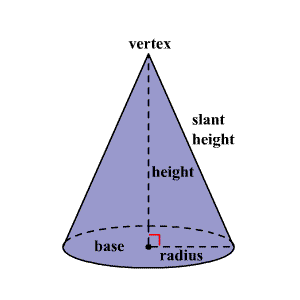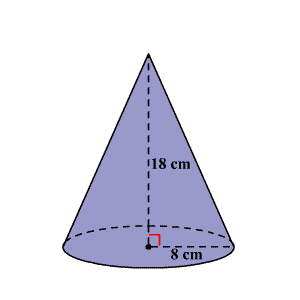# Volume of a Cone

A cone is a three-dimensional figure with one circular base. A curved surface connects the base and the vertex.The volume of a $3$ -dimensional solid is the amount of space it occupies.  Volume is measured in cubic units ( ${\text{in}}^{3},{\text{ft}}^{3},{\text{cm}}^{3},{\text{m}}^{3},$ et cetera).  Be sure that all of the measurements are in the same unit before computing the volume.

The volume $V$ of a cone with radius $r$ is one-third the area of the base $B$ times the height $h$ .

$V=\frac{1}{3}Bh\text{\hspace{0.17em}}\text{\hspace{0.17em}}\text{or}\text{\hspace{0.17em}}\text{\hspace{0.17em}}V=\frac{1}{3}\pi {r}^{2}h,\text{\hspace{0.17em}}\text{\hspace{0.17em}}\text{where}\text{\hspace{0.17em}}\text{\hspace{0.17em}}B=\pi {r}^{2}$

Note : The formula for the volume of an oblique cone is the same as that of a right one.

The volumes of a cone and a cylinder are related in the same way as the volumes of a pyramid and a prism are related. If the heights of a cone and a cylinder are equal, then the volume of the cylinder is three times as much as the volume of a cone.

Example:

Find the volume of the cone shown. Round to the nearest tenth of a cubic centimeter.Solution

From the figure, the radius of the cone is $8$ cm and the height is $18$ cm.

The formula for the volume of a cone is,

$V=\frac{1}{3}\pi {r}^{2}h$

Substitute $8$ for $r$ and $18$ for $h$ .

$V=\frac{1}{3}\pi {\left(8\right)}^{2}\left(18\right)$

Simplify.

$\begin{array}{l}V=\frac{1}{3}\pi \left(64\right)\left(18\right)\\ =384\pi \\ \approx 1206.4\end{array}$

Therefore, the volume of the cone is about $1206.4$ cubic centimeters.